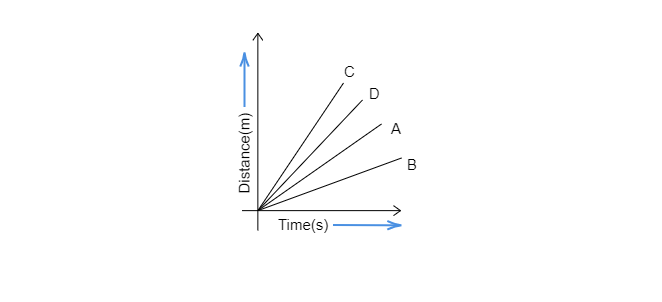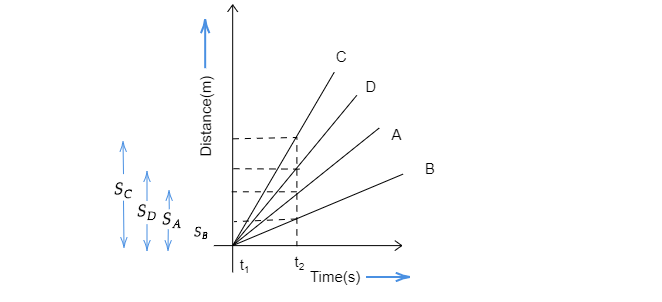$(a)$. Car A is faster than car D.
$(b)$. Car B is the slowest.
$(c)$. Car D is faster than car C.
$(d)$. Car C is the slowest." ">

# Four cars A, B, C, and D are moving on a leveled road. Their distance versus time graphs is shown in the figure. Choose the correct statement$(a)$. Car A is faster than car D.$(b)$. Car B is the slowest.$(c)$. Car D is faster than car C.$(d)$. Car C is the slowest."In the same time interval $(t_2-t_1)$, Distances covered by cars A, B, C and D are $S_A,\ S_B,\ S_C$ and $S_D$.

And in the above graph, it is clear that $S_B So, Car C is moving fastest and car B is moving slowest. From the given graph we can conclude that car B is the slowest. So, option$(b)\$. is correct.

Updated on: 10-Oct-2022

78 Views